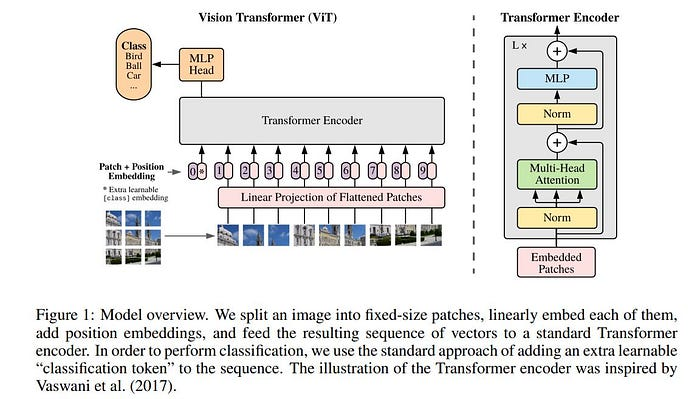# CV002-CNN vs. Vision Transformer

Article Outline

Transformers由于其计算效率和可扩展性而成为NLP中的首选模型。在计算机视觉中，卷积神经网络（CNN）架构仍然占主导地位，但一些研究人员已经尝试将自注意相结合。作者尝试将标准Transformer直接应用于图像，并发现当在中等大小的数据集上训练时，与类似ResNet的架构相比，模型的准确性适中。然而，当在更大的数据集上训练时，ViT取得了更优异的结果，并在多个图像识别基准上接近或超过了现有技术。# Example: CNN vs. Vision Transformer

``````from kaggle.api.kaggle_api_extended import KaggleApi

api = KaggleApi()
api.authenticate()

# we write to the current directory with './'

``````!unzip -qq kaggle-cat-vs-dog-dataset.zip
!rm -r kaggle-cat-vs-dog-dataset.zip``````

``````!git clone https://github.com/RustamyF/vision-transformer.git
!mv vision-transformer/vision_tr .``````

``````import torch.nn as nn
import torch
import torch.optim as optim

from torchvision import datasets, models, transforms
from PIL import Image
from sklearn.model_selection import train_test_split

import os

def __init__(self):
self.cat_path = 'kagglecatsanddogs_3367a/PetImages/Cat'
self.dog_path = 'kagglecatsanddogs_3367a/PetImages/Dog'

def delete_non_jpeg_files(self, directory):
for filename in os.listdir(directory):
if not filename.endswith('.jpg') and not filename.endswith('.jpeg'):
file_path = os.path.join(directory, filename)
try:
elif os.path.isdir(file_path):
shutil.rmtree(file_path)
print('deleted', file_path)
except Exception as e:
print('Failed to delete %s. Reason: %s' % (file_path, e))

def data(self):
self.delete_non_jpeg_files(self.dog_path)
self.delete_non_jpeg_files(self.cat_path)

dog_list = os.listdir(self.dog_path)
dog_list = [(os.path.join(self.dog_path, i), 1) for i in dog_list]

cat_list = os.listdir(self.cat_path)
cat_list = [(os.path.join(self.cat_path, i), 0) for i in cat_list]

total_list = cat_list + dog_list

train_list, test_list = train_test_split(total_list, test_size=0.2)
train_list, val_list = train_test_split(train_list, test_size=0.2)
print('train list', len(train_list))
print('test list', len(test_list))
print('val list', len(val_list))
return train_list, test_list, val_list

# data Augumentation
transform = transforms.Compose([
transforms.Resize((224, 224)),
transforms.RandomResizedCrop(224),
transforms.RandomHorizontalFlip(),
transforms.ToTensor(),
])

class dataset(torch.utils.data.Dataset):

def __init__(self, file_list, transform=None):
self.file_list = file_list
self.transform = transform

# dataset length
def __len__(self):
self.filelength = len(self.file_list)
return self.filelength

# load an one of images
def __getitem__(self, idx):
img_path, label = self.file_list[idx]
img = Image.open(img_path).convert('RGB')
img_transformed = self.transform(img)
return img_transformed, label``````

## *CNN 方法 *

``````class Cnn(nn.Module):
def __init__(self):
super(Cnn, self).__init__()

self.layer1 = nn.Sequential(
nn.BatchNorm2d(16),
nn.ReLU(),
nn.MaxPool2d(2)
)

self.layer2 = nn.Sequential(
nn.BatchNorm2d(32),
nn.ReLU(),
nn.MaxPool2d(2)
)

self.layer3 = nn.Sequential(
nn.BatchNorm2d(64),
nn.ReLU(),
nn.MaxPool2d(2)
)

self.fc1 = nn.Linear(3 * 3 * 64, 10)
self.dropout = nn.Dropout(0.5)
self.fc2 = nn.Linear(10, 2)
self.relu = nn.ReLU()

def forward(self, x):
out = self.layer1(x)
out = self.layer2(out)
out = self.layer3(out)
out = out.view(out.size(0), -1)
out = self.relu(self.fc1(out))
out = self.fc2(out)
return out``````

## VIT方法

VIT架构采用可定制的尺寸设计，可根据特定要求进行调整。对于这种大小的图像数据集，这种架构仍然很大。

``````from vision_tr.simple_vit import ViT
model = ViT(
image_size=224,
patch_size=32,
num_classes=2,
dim=128,
depth=12,
mlp_dim=1024,
dropout=0.1,
emb_dropout=0.1,
).to(device)``````

VIT中的每个参数都起着关键作用，如下所述：

• image_size=224：此参数指定模型的输入图像的所需大小（宽度和高度）。在这种情况下，期望图像的大小为224x224像素。
• patch_size=32：图像被划分为较小的patch，此参数定义每个patch的大小（宽度和高度）。在这种情况下，每个patch都是32x32像素。
• num_classes=2：该参数表示分类任务中的类数。在这个例子中，模型被设计为将输入分为两类（猫和狗）。
• dim=128：它指定了模型中嵌入向量的维度。嵌入捕获每个图像patch的表示。
• depth=12：此参数定义VIT（编码器模型）中的深度或层数。更高的深度允许更复杂的特征提取。